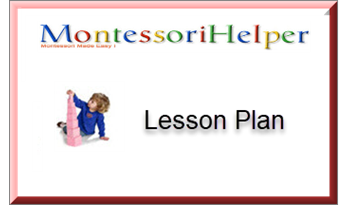## MATERIALS

Box 5 is a triangular box  which contains 8 blue triangles:
2 small equilateral triangles.
1 small obtuse angle scalene triangle.
2 large right angle isosceles triangles.
1 small right angle scalene triangle.
2 medium right angle scalene triangles.

## DIRECT AIM

To give the child the opportunity to experiment with various geometric shapes.

## INDIRECT AIM

To show that all plane, flat, rectilinear figures are composed of triangles.
To indirectly prepare the child for later work in Maths and Geometry.

## AGE

4 to 4.5 years approx.

+ +